# Beginning Algebra Tutorial 28

Beginning Algebra
Tutorial 28: Multiplying PolynomialsLearning Objectives

After completing this tutorial, you should be able to:
1. Multiply any polynomial times any other polynomial.
2. Use the FOIL method to multiply a binomial times a binomial.Introduction

In this tutorial we help you expand your knowledge of polynomials by looking at multiplying polynomials together.  We will look at using the distributive property, initially shown in Tutorial 8: Properties of Real Numbers, to help us out.  Again, we are using a concept that you have already seen to apply to the new concept.  After going through this tutorial you should have multiplying polynomials down pat.Tutorial

Multiplying Polynomials

In general, when multiplying two polynomials together, use the distributive property, initially shown in Tutorial 8: Properties of Real Numbers,  until every term of one polynomial is multiplied times every term of the other polynomial.  Make sure that you simplify your answer by combining any like terms.

On this page we will look at some of the more common types of polynomials to illustrate this idea.

(Monomial)(Monomial)

In this case, there is only one term in each polynomial.  You simply multiply the two terms together.Example 1:   Find the following product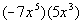.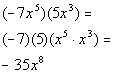(Monomial)(Polynomial)

In this case, there is only one term in one polynomial and more than one term in the other.  You need to distribute the monomial to EVERY term of the other polynomial.Example 2:   Find the following product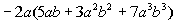.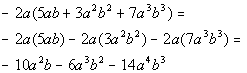*Dist. -2a

(Binomial)(Binomial)

In this case, both polynomials have two terms.  You need to distribute both terms of one polynomial times both terms of the other polynomial.

One way to keep track of your distributive property is to
Use the FOIL method.   Note that this method only works on (Binomial)(Binomial).

F First terms O Outside terms I Inside terms L Last terms

This is a fancy way of saying take every term of the first binomial times every term of the second binomial.  In other words, do the distributive  property for every term in the first binomial.Example 3:  Find the following product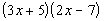.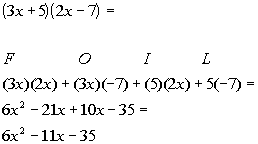*Use the FOIL method

*Combine like termsExample 4:   Find the following product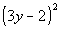.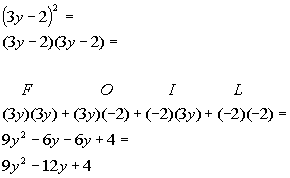*Rewrite as base
(3y - 2) times itself

*Use the FOIL method

*Combine like terms

(Polynomial)(Polynomial)

As mentioned above, use the distributive property until every term of one polynomial is multiplied times every term of the other polynomial.  Make sure that you simplify your answer by combining any like terms.Example 5:   Find the following product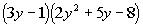.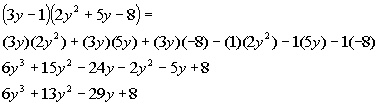*Use Dist. Prop. twice

*Combine like termsPractice Problems

These are practice problems to help bring you to the next level.  It will allow you to check and see if you have an understanding of these types of problems. Math works just like anything else, if you want to get good at it, then you need to practice it.  Even the best athletes and musicians had help along the way and lots of practice, practice, practice, to get good at their sport or instrument.  In fact there is no such thing as too much practice.

To get the most out of these, you should work the problem out on your own and then check your answer by clicking on the link for the answer/discussion for that  problem.  At the link you will find the answer as well as any steps that went into finding that answer.Practice Problems 1a - 1d: Find the following products.

1a.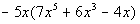1b.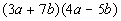1c.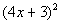1d.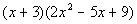Need Extra Help on these Topics?

The following are webpages that can assist you in the topics that were covered on this page:

http://www.algebrahelp.com/lessons/simplifying/distribution/
This website helps with the distributive property.

http://www.algebrahelp.com/lessons/simplifying/foilmethod/
This website helps with the FOIL method and (polynomial)(polynomial).

http://www.purplemath.com/modules/polymult.htm
This webpage helps with multiplying polynomials.

Go to Get Help Outside the Classroom found in Tutorial 1: How to Succeed in a Math Class for some more suggestions.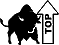Last revised on August 2, 2011 by Kim Seward.